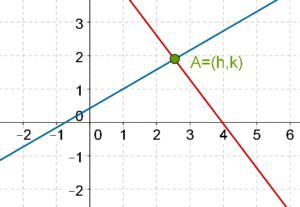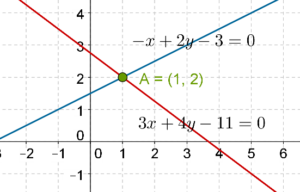# Graphically Solving a Pair of Linear Equations

Go back to  'Pair of Linear Equations in Two Variables'

We have seen that for any linear equation, the graph we get is a straight line. This means that the coordinates of every point on this line will satisfy the linear equation. Now, suppose that we take a pair of linear equations:

$\begin{array}{l}{a_1}x + {b_1}y + {c_1} = 0\\{a_2}x + {b_2}y + {c_2} = 0\end{array}$.

Suppose that we draw the lines corresponding to both these equations on common axes, and obtain the following graph:What is the geometrical interpretation of this? The two lines intersect at the point A. Clearly, since a lies on both the lines, the coordinates of A, that is, $$\left( {h,\;k} \right)$$, will satisfy both the linear equations. Thus,

$\begin{array}{l}{a_1}h + {b_1}k + {c_1} = 0\\{a_2}h + {b_2}k + {c_2} = 0\end{array}$

Technically speaking, we will say that the coordinates of A form the solution to the pair of linear equations given by (1). Sometimes, we might also omit the phrase “coordinates of” and simply state: A is a solution to the pair of linear equations given by (1). It should be understood that by A, we mean the coordinates of A.

As an example, consider the following pair of linear equations:

$\begin{array}{l} - x + 2y - 3 = 0\\ 3x + 4y - 11 = 0\end{array}$

We draw the corresponding lines on the same axes:The point of intersection is $$A\left( {1,\,\,2} \right)$$, which means that $$x = 1,\;\;y = 2$$ is a solution to the pair of linear equations given by (2). In fact, it is the only solution to the pair, as two non-parallel lines cannot intersect in more than one point.

Pair of Linear Equations in 2 Variables
Pair of Linear Equations in 2 Variables
grade 10 | Questions Set 2
Pair of Linear Equations in 2 Variables
Pair of Linear Equations in 2 Variables
grade 10 | Questions Set 1